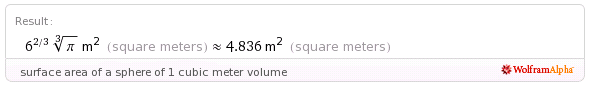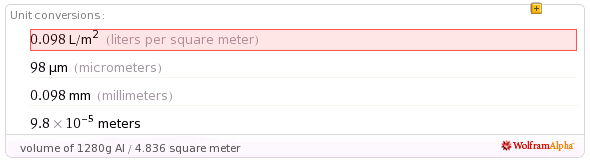# Wishful Coding

Didn't you ever wish your computer understood you?

## Vacuum Balloon

You know these helium balloons? They float because helium is lighter, less dense then air. You know what is even lighter than air? No air at all!

I came across the idea on this website, where he notes

I haven’t done any math, but I assume this isn’t feasible at the present time.

I have done some math, or rather, asked Wolfram Alpha.

So, first of all, how heavy is a cubic meter of air anyaway?Ok, so our balloon can weigh just over a kilo and still float. But what is the surface area of a balloon of that size?Almost five square meter. So with one kilo of matter, we need to make a balloon of nearly 5 square meter surface area.Aww, not looking good. If we use aluminum1, we can make a shell of a tenth of a millimeter thick. This shell will have to resist 1 bar, which equals 10 newton per square centimeter.I just picked this up from Wikipedia, but presumably this means the stress on the shell will be 3162 newton per square millimeter.

Now, remember that this is a vacuum, so while a pressurized balloon retains it shape because of the pressure, the biggest problem with our vacuum balloon is the buckling force.

The smallest dent, a gust of wind, or even gravity itself, will cause the balloon to implode. I asked my dad2, and he asked his engineer, how to calculate this force. Third order magic.

1. We should be using carbon or some composite probably, but it doesn’t really get much beyond 1mm anyway.

2. He’s an architect.